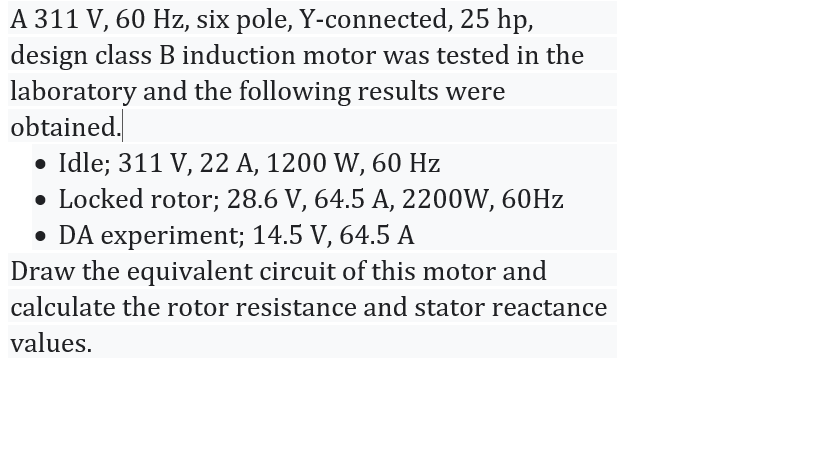A $$311 \mathrm{~V}, 60 \mathrm{~Hz}$$, six pole, Y-connected, $$25 \mathrm{hp}$$, design class B induction motor was tested in the laboratory and the following results were obtained.

- Idle; $$311 \mathrm{~V}, 22 \mathrm{~A}, 1200 \mathrm{~W}, 60 \mathrm{~Hz}$$

- Locked rotor; $$28.6 \mathrm{~V}, 64.5 \mathrm{~A}, 2200 \mathrm{~W}, 60 \mathrm{~Hz}$$

- DA experiment; $$14.5 \mathrm{~V}, 64.5 \mathrm{~A}$$ Draw the equivalent circuit of this motor and calculate the rotor resistance and stator reactance values.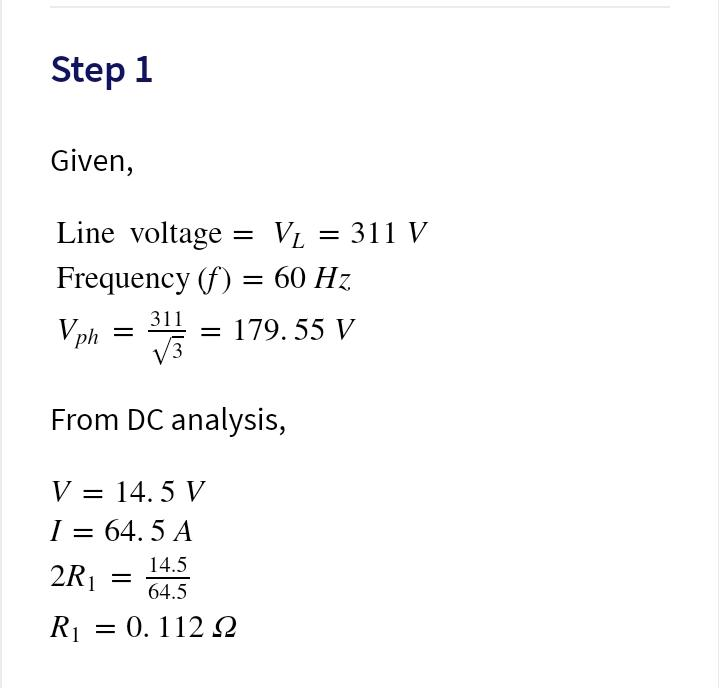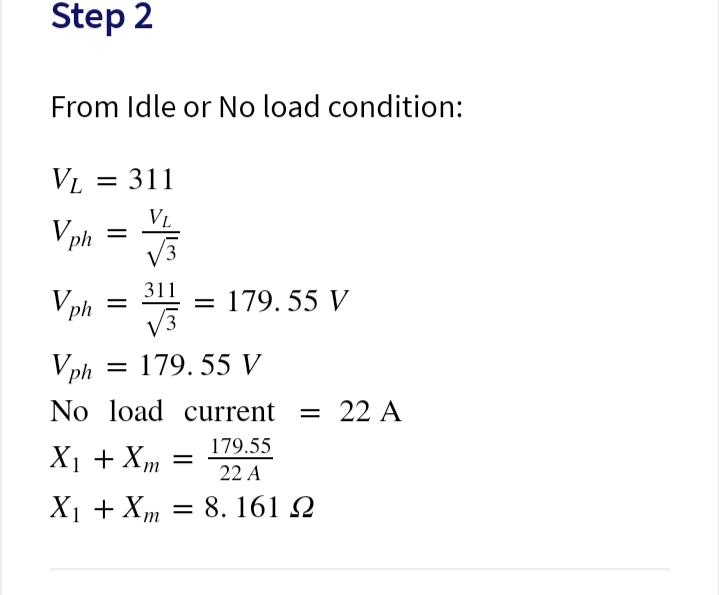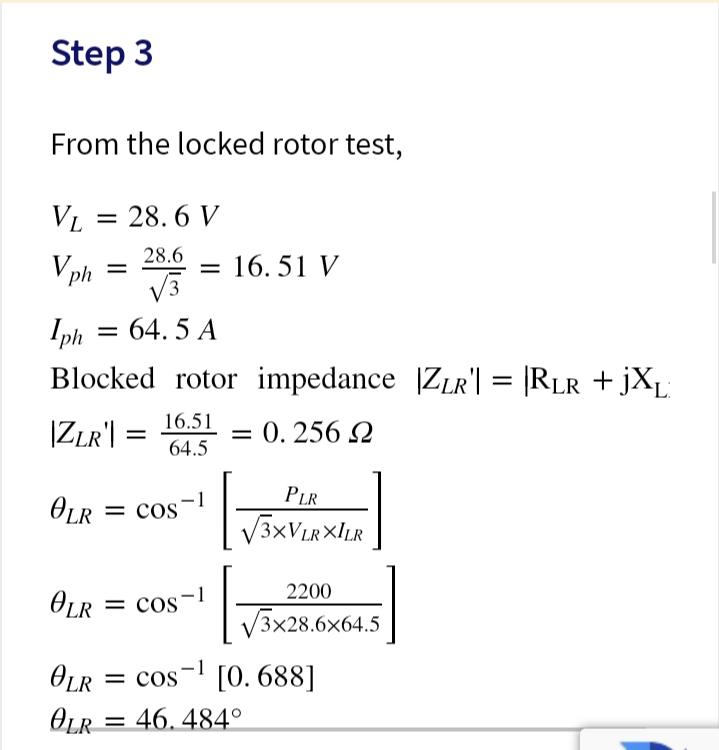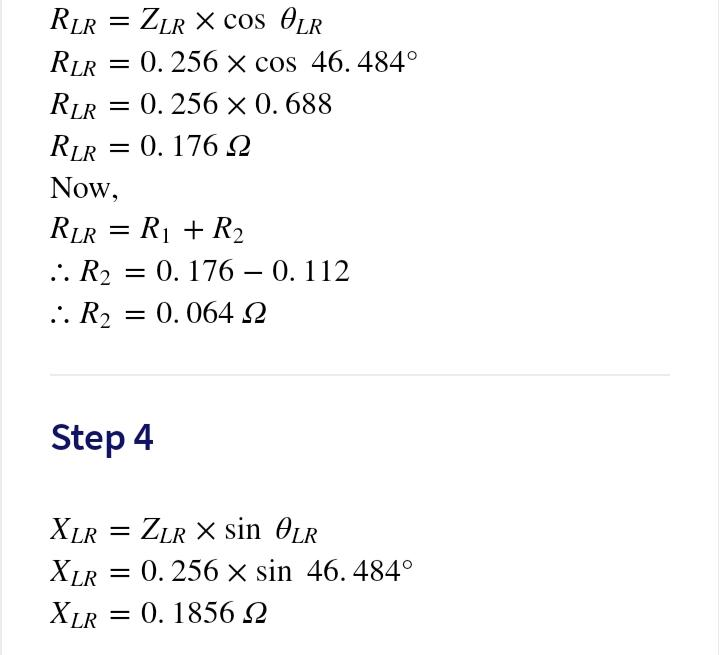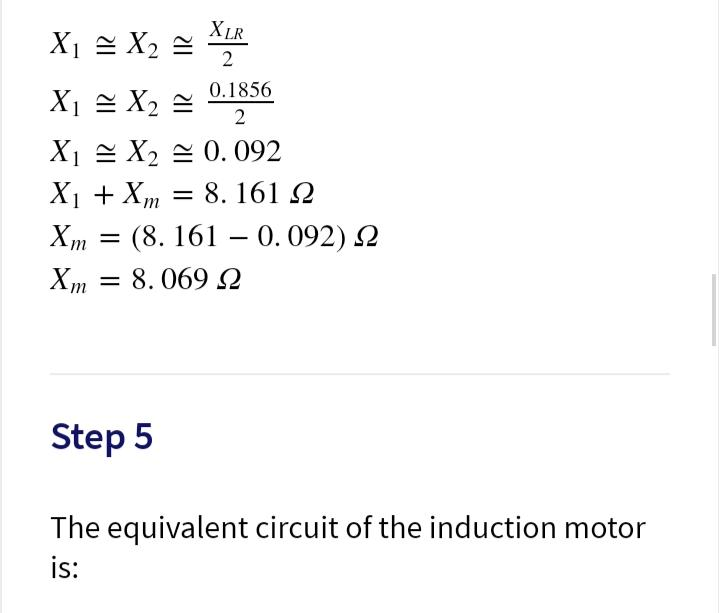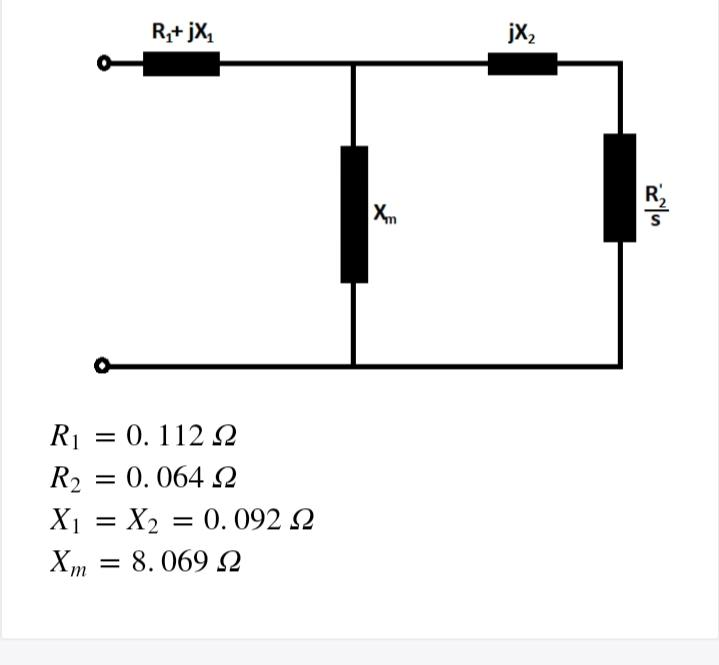Similar Homework Help Questions
• ### A 208-V, six-pole, Y-connected, 25-hp design class B induction motor is tested in the laboratory, with...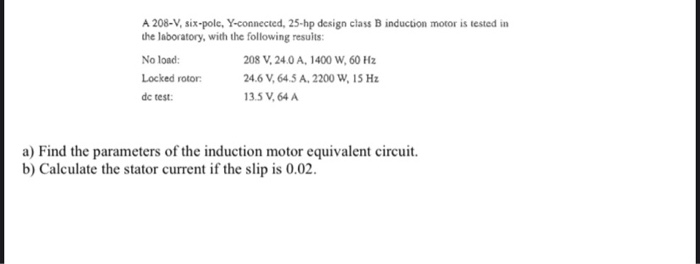A 208-V, six-pole, Y-connected, 25-hp design class B induction motor is tested in the laboratory, with the following results: No load: 208 V, 24.0 A, 1400 W, 60 Hz Locked rotor: 24.6 V, 64.5 A. 2200 W, 15 Hz 13.5 V, 64 A de test: a) Find the parameters of the induction motor equivalent circuit. b) Calculate the stator current if the slip is 0.02.

• ### Name: Student ID: A 208-V, six-pole, Y-connected, 25-hp design class B induction motor is tested in...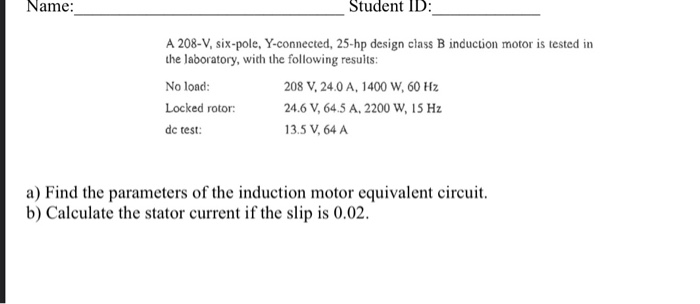Name: Student ID: A 208-V, six-pole, Y-connected, 25-hp design class B induction motor is tested in the laboratory, with the following results: No load: 208 V, 24.0 A, 1400 W, 60 Hz Locked rotor: 24.6 V, 64.5 A, 2200 W, 15 Hz dc test: 13.5 V, 64 A a) Find the parameters of the induction motor equivalent circuit. b) Calculate the stator current if the slip is 0.02.

• ### 4) A 208V, 60 Hz, 6 pole, Y-connected, 25 hp induction motor is tested in the laboratory with the...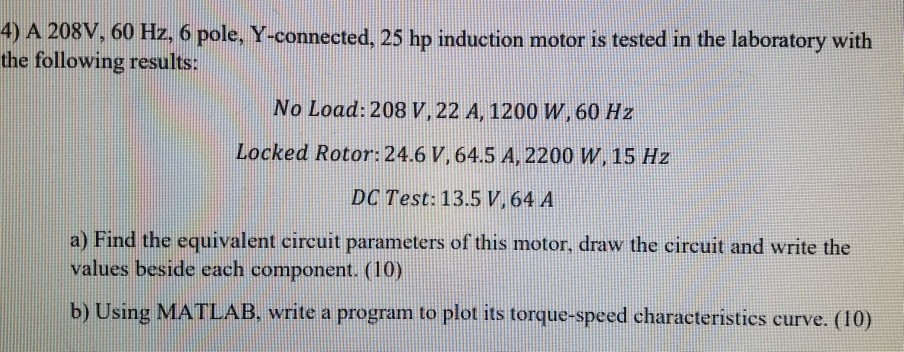please show psuedocode 4) A 208V, 60 Hz, 6 pole, Y-connected, 25 hp induction motor is tested in the laboratory with the following results: No Load: 208 V,22 A, 1200 W,60 Hz Locked Rotor: 24.6 V,64.5 4,2200 W.15 Hz DC Test: 13.5 V.64 4 a) Find the equivalent circuit parameters of this motor, draw the circuit and write the values beside each component. (10) b) Using MATLAB, write a program to plot its torque-speed characteristics curve. (10) 4) A 208V,...

• ### III A 208-V, 60 Hz, six-pole Y-connected 25-hp design class B induction motor is tested in...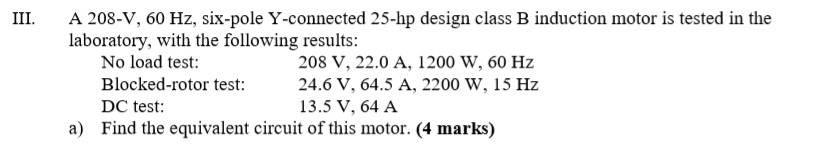III A 208-V, 60 Hz, six-pole Y-connected 25-hp design class B induction motor is tested in the laboratory, with the following results: No load test: Blocked-rotor test: DC test: 208 V, 22.0 A, 1200 W, 60 Hz 24.6 V, 64.5 A, 2200 W, 15 Hz 13.5 V, 64 A a) Find the equivalent cireuit of this motor. (4 marksy)

• ### please urgent electrical or mechanical engineer can help? I have 20 hours.I have to finish. Pleaseeee :(

Question 1) A $$380 \mathrm{~V} 60 \mathrm{~Hz}$$ two-pole three-phase asynchronous motor has a reverse power factor of 0.80. Stator resistance $$0,200 \Omega$$ rotor resistance $$0,120 \Omega$$ iron reactance resistance $$150 \Omega$$ friction andwind losses $$450 \mathrm{~W}$$, iron losses $$1500 \mathrm{~W}$$ and stator copper power loss $$1215 \mathrm{~W}$$. If the slip is $$5 \%,$$ calculate the efficiency of the motor and the shaft moment.Question 2) Three synchronous generators feed 150 of the motors given in the first question. The idle operating...

• ### Problem 2 (10 points): A 3-phase, 208-V (H), six-pole Y-connected, 25-hp, design class B (X,-15%)...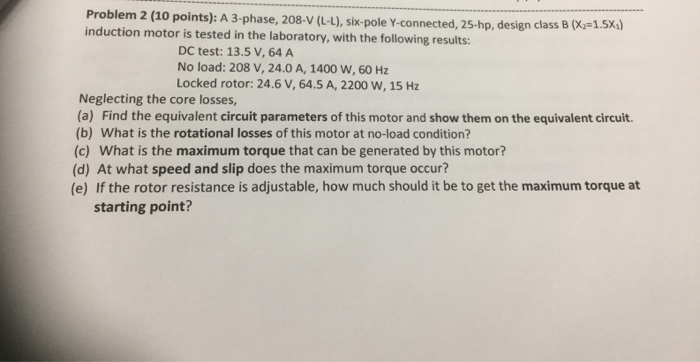Problem 2 (10 points): A 3-phase, 208-V (H), six-pole Y-connected, 25-hp, design class B (X,-15%) induction motor is tested in the laboratory, with the following results: DC test: 13.5 V, 64 A No load: 208 V, 24.0 A, 1400 W, 60 Hz Locked rotor: 24.6 V, 64.5 A, 2200 W, 15 Hz Neglecting the core losses (a) Find the equivalent circuit parameters of this motor and show them on the equivalent circuit. (b) What is the rotational losses of this...

• ### :00 Hz, six-pole Y-connected 40-hp design class B induction motorX -0.4Xux, and 3. A 460-V. 60Hz,...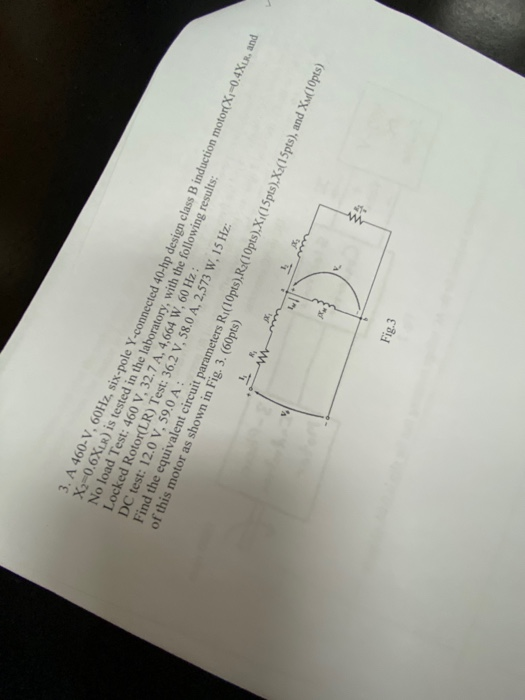:00 Hz, six-pole Y-connected 40-hp design class B induction motorX -0.4Xux, and 3. A 460-V. 60Hz, six-pole Y-connected 40-hp X2-0.6XLR) is tested in the laboratory, with the following results: No load Test: 460 V, 32.7 A, 4,664 W, 60 Hz Locked Rotor(LR) Test: 36.2 V, 58.0 A, 2,573 W, 15 Hz: DC test: 12.0 V, 59.0 A: Find the equivalent circuit parameters Ri(10pts),R (10pts),X (15pts),X:(15pts), and Xx(10pts) of this motor as shown in Fig. 3. (60pts) Fig. 3

• ### Name: 23. A 3-phase, 5000 hp, 6000 v,60 Hz, 12-pole wound-rotor induction motor has the 1, resistance between stator terminals-o12 Ω 2. resistance between rotor slip-rings-0.0073 Ω 3. windage and...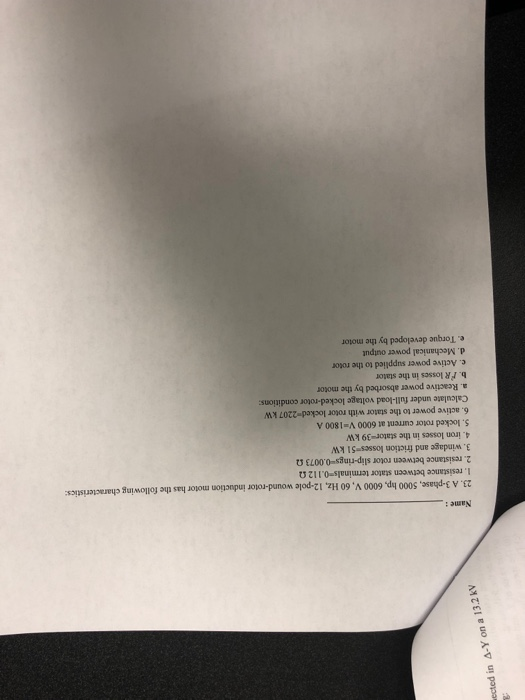Name: 23. A 3-phase, 5000 hp, 6000 v,60 Hz, 12-pole wound-rotor induction motor has the 1, resistance between stator terminals-o12 Ω 2. resistance between rotor slip-rings-0.0073 Ω 3. windage and friction losses-51 kW following characteristics 4. iron losses in the stator-39 kW 5. locked rotor current at 6000 V-1800 A 6. active power to the stator with rotor locked 2207 kw Calculate under full-load voltage locked-rotor conditions a. Reactive power absorbed by the motor b. FR losses in the stator...

• ### 2) A 460 V, 4-pole, 60 Hz, Y-connected, 40 hp, design class A induction motor is tested with the following results: No-load: 460 V, 8.7 A, 2500 W. 60 Hz Locked rotor: 42 V.48 A, 31 10 W. 12 Hz DC tes...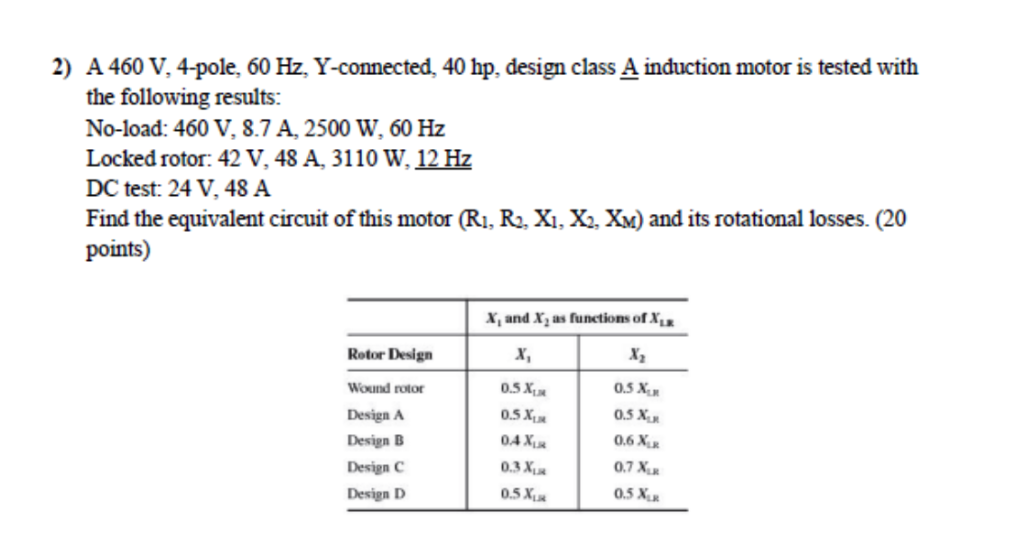2) A 460 V, 4-pole, 60 Hz, Y-connected, 40 hp, design class A induction motor is tested with the following results: No-load: 460 V, 8.7 A, 2500 W. 60 Hz Locked rotor: 42 V.48 A, 31 10 W. 12 Hz DC test: 24 V, 48 A Find the equivalent circuit of this motor (Ri, Ro. Xi. X. XM) and its rotational losses. (20 points) X, and A2 as functions of XL Retor Design Wound rotor Design A Design B Design...

• ### A 240 V, 50 Hz, six-pole Y-connected, 18 kW, induction motor is tested in the laboratory,...

A 240 V, 50 Hz, six-pole Y-connected, 18 kW, induction motor is tested in the laboratory, with the following results: No load test: Terminal voltage: 240 V, phase current: 6.0 A, AC supply frequency: 50 Hz Locked rotor: Terminal voltage: 36 V, phase current: 8.5 A, input active power: 235 W, AC supply frequency: 50 Hz DC test: DC voltage: 13.5 V, DC current: 10.5 A Find the equivalent circuit parameters of this motor.

Free Homework App